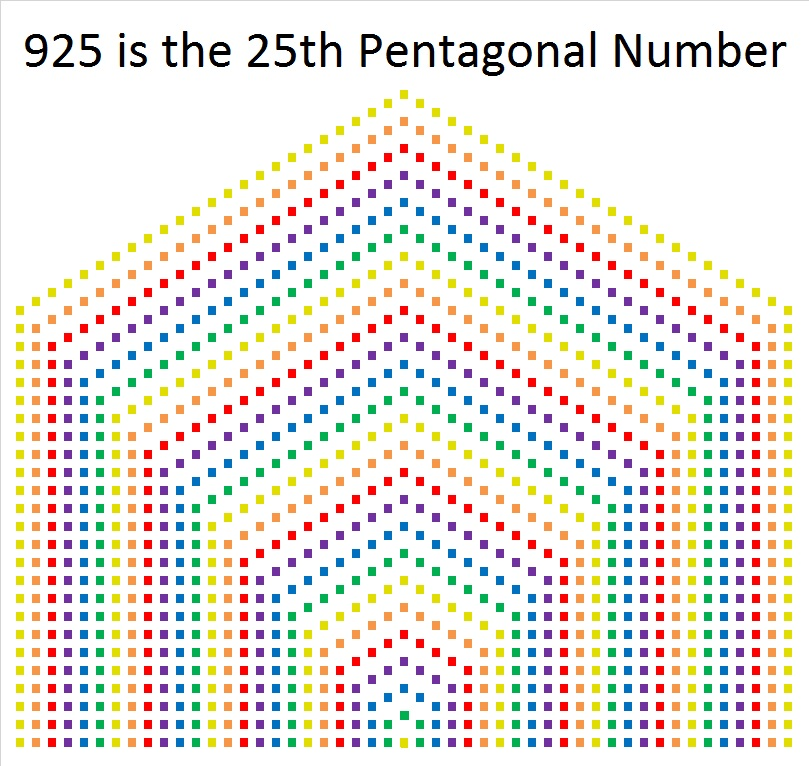# 1513 is the Sum of Squares

### Today’s Puzzle:

How can you arrange 1513 dots into a perfect square when √1513 is irrational?

The answer is you arrange the dots into a centered square like this:You can arrange them like that because 1513 is the sum of consecutive squares.

### Factors of 1513:

• 1513 is a composite number.
• Prime factorization: 1513 = 17 × 89
• 1513 has no exponents greater than 1 in its prime factorization, so √1513 cannot be simplified.
• The exponents in the prime factorization are 1, and 1. Adding one to each exponent and multiplying we get (1 + 1)(1 + 1) = 2 × 2 = 4. Therefore 1513 has exactly 4 factors.
• The factors of 1513 are outlined with their factor pair partners in the graphic below.### More about the Number 1513:

1513 is the sum of two squares in two different ways:
28² + 27²  = 1513, and
37² + 12²  = 1513.

1513 is the hypotenuse of FOUR Pythagorean triples:
55-1512-1513,  calculated from 28² – 27², 2(28)( 27), 28² + 27²
663-1360-1513, which is 17 times (39-80-89)
712-1335-1513, which is (8-15-17) times 89
888-1225-1513, calculated from 2(37)(12), 37² – 12², 37² + 12²

### Could 1513 be a prime number?

Since its last two digits divided by 4 leave a remainder of 1, and 28² + 27² = 1513 with   28 and 27 having no common prime factors, 1513 will be prime unless it is divisible by a prime number Pythagorean triple hypotenuse less than or equal to √1513. Is 1513 divisible by 5, 13, 17, 29, or 37? Yes, it is divisible by 17, so 1513 is NOT a prime number.

37² + 12²  = 1513 and 37 and 12 have no common prime factors, so we could have arrived at the same result using those numbers.

Note: Numbers that are the sum of two squares in two or more ways are never prime.

# What Kind of Shape is 925 In?

925 is the 22nd Centered Square number because 22² + 21² = 925. I made this graphic to show this fact through the use of color. Look at the center of the centered square. Can you see how the single yellow square and the four small green squares in the center correspond to the same colored squares in the smaller squares? The pattern continues from the inside of the centered square to the outside.925 is the sum of two squares these THREE ways:

• 22² + 21² = 925
• 27² + 14² = 925
• 30² + 5² = 925

925 is the hypotenuse of SEVEN Pythagorean triples:

• 43-924-925
• 259-888-925
• 285-880-925
• 300-875-925
• 520-765-925
• 533-756-925
• 555-740-925

925 is the 25th pentagonal number because 3((25²) – 25)/2 = 925. The shape in the graphic below of 925 tiny squares may look more like a house, but it is still very much a pentagon.925 looks interesting in a few other bases:

4141 in BASE 6
1K1 BASE 22 (K is 20 in base 10)
151 BASE 28
PP in BASE 36 (P is 25 in base 10)

• 925 is a composite number.
• Prime factorization: 925 = 5 × 5 × 37, which can be written 925 = 2² × 37
• The exponents in the prime factorization are 2 and 1. Adding one to each and multiplying we get (2 + 1)(1 + 1) = 3 × 2  = 6. Therefore 925 has exactly 6 factors.
• Factors of 925: 1, 5, 25, 37, 185, 925
• Factor pairs: 925 = 1 × 925, 5 × 185, or 25 × 37
• Taking the factor pair with the largest square number factor, we get √925 = (√25)(√37) = 5√37 ≈ 30.41381